#### How To Calculate Variation Ratio Test (aka. F-Ratio test)?

If we take samples from two populations and wish to ascertain whether the variations of both samples are equal, we can use the Variation Ratio test also known as the F-Ratio test to determine this.

We will need to activate the Analysis ToolPak in Excel to access the F-test function.

To run the F-test we will use sample data file Variation Ratio Test sample data.

## Adding the Analysis ToolPak to the Data tab

If the Analysis section on the Data tab is visible, you can skip theses steps (see screenshot).

If the Analysis section is not activated, follow these steps to activate the Analysis ToolPak.

1. In Excel, click File.

2. Click Options.

3. In the Excel Options window click Add-ins, in Manage select Excel Add-ins, click Go.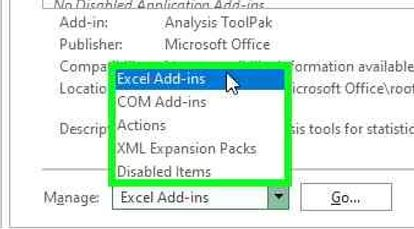4. The Add-ins window opens, select Analysis ToolPak and click OK.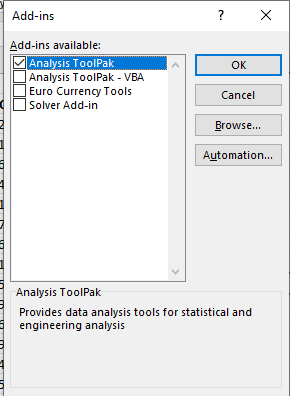5. The Analysis section is now active on the Data tab.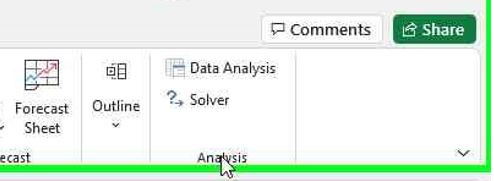## Running the Variation Ratio / F-Test

1. Open the Variation Ratio Test sample data spreadsheet.

2. On the Data tab, click Data Analysis, then select F-test Two-Sample for Variances, click OK.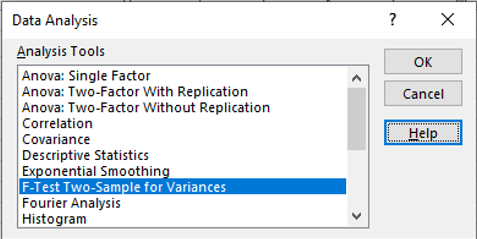3. The F-test Two-Sample for Variances, window opens, click the Variable 1 Range arrow.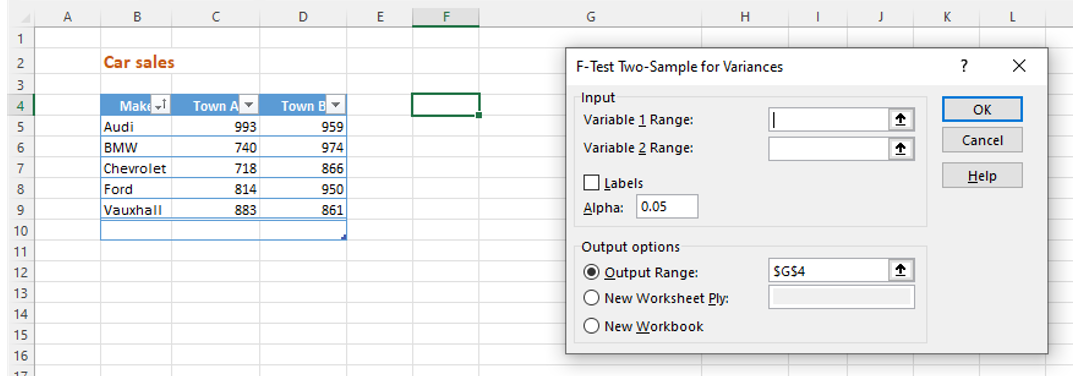4. The window contracts to the Variable 1 Range field, select C4-C9, click the arrow again.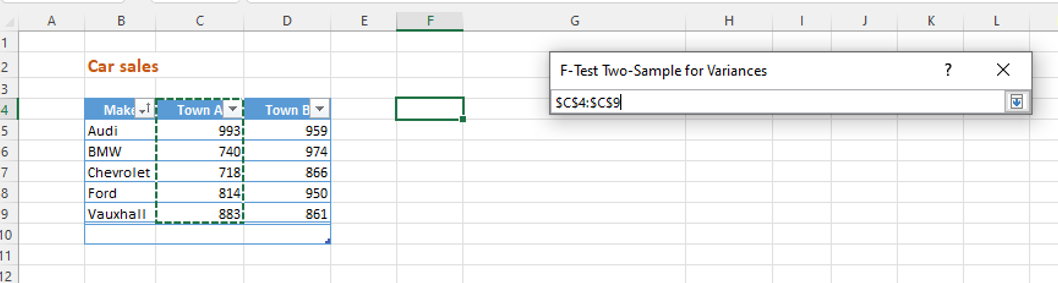5. Click the Variable 2 Range arrow, select D4-D9, click the arrow again.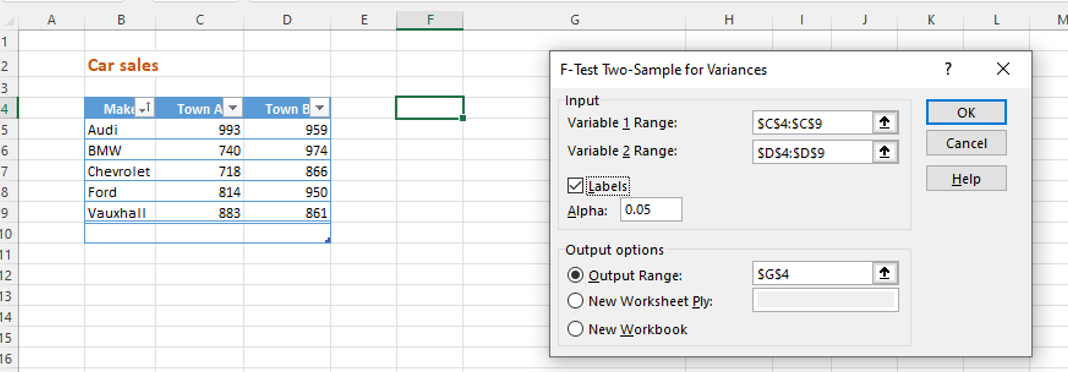6. Click Labels. In Output options click the Output Range arrow.

7. Select Cell F4, click the arrow again, click OK.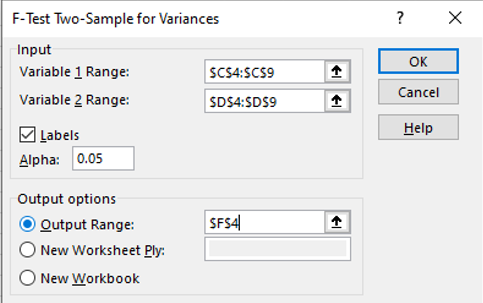8. Excel displays the F-test results.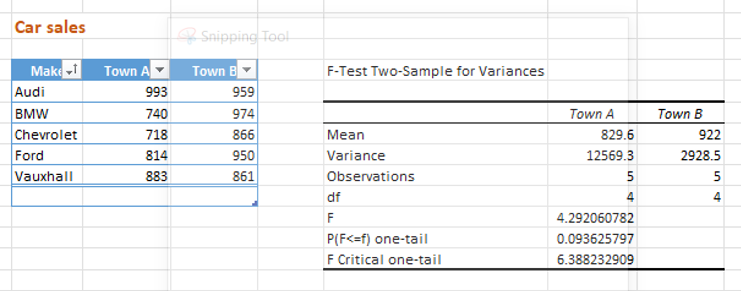## Quick reference guide

### Steps to add the Analysis ToolPak

1. In Excel, click File.
2. Click Options.
3. In the Excel Options window click Add-ins, in Manage select Excel Add-ins, click Go.
4. The Add-ins window opens, select Analysis ToolPak and click OK.
5. The Analysis section is now active on the Data tab.

## Running the Variation Ratio / F-Test

1. Open the spreadsheet with the data you wish to analyse.
2. On the Data tab, click Data Analysis, then select F-test Two-Sample for Variances, click OK.
3. The F-test Two-Sample for Variances, window opens, click the Variable 1 Range arrow.
4. The window contracts to the Variable 1 Range field, select the first variable range, click the arrow again.
5. Click the Variable 2 Range arrow, select the first variable range, click the arrow again.
6. Click Labels. In Output options click the Output Range arrow.
7. Select the cell where you want the results to appear, click the arrow again, click OK.8. Excel displays the F-test results.

## Using F.TEST function

To calculate the variation ratio test in Excel, you can use the F.TEST function. The syntax for this function is as follows:

F.TEST(array1,array2,tails,type)

where:

• array1 and array2 are the two arrays of data you want to compare
• tails specifies the number of tails for the test (either 1 or 2)
• type specifies the type of F-test to perform (either 1 or 2)

Here is an example of how to use the F.TEST function to perform a variation ratio test in Excel:

1. Enter your data into two separate columns in an Excel worksheet, with the first column representing one sample and the second column representing the other sample.
2. Select a cell where you want to display the result of the F-test.
3. Type the following formula: =F.TEST(A1:A10,B1:B10,2,2), where A1:A10 and B1:B10 are the ranges of the two sample data.
4. Press enter to calculate the F-ratio.